# NCERT Exemplar Class 12 Physics Solutions for Chapter 5 Magnetism And Matter

NCERT Exemplar Solutions for Class 12 Physics Chapter 5 Magnetism and Matter not only serve as merely a question bank for examinations but are primarily meant to improve the quality of the teaching/learning process in schools. Students can benefit from these solutions by referring to them while solving the exercise questions given in the textbook for self-assessment and also in mastering the basic techniques of problem-solving.

NCERT Exemplar for Class 12 Physics Chapter 5 provides you with an opportunity to get well-versed in the topics covered in Chapter 5 through different kinds of questions that is usually of advanced level and are asked in the national level entrance and competitive examinations.

## Download the PDF of NCERT Exemplar Solutions for Class 12 Physics Chapter 5 Magnetism and Matter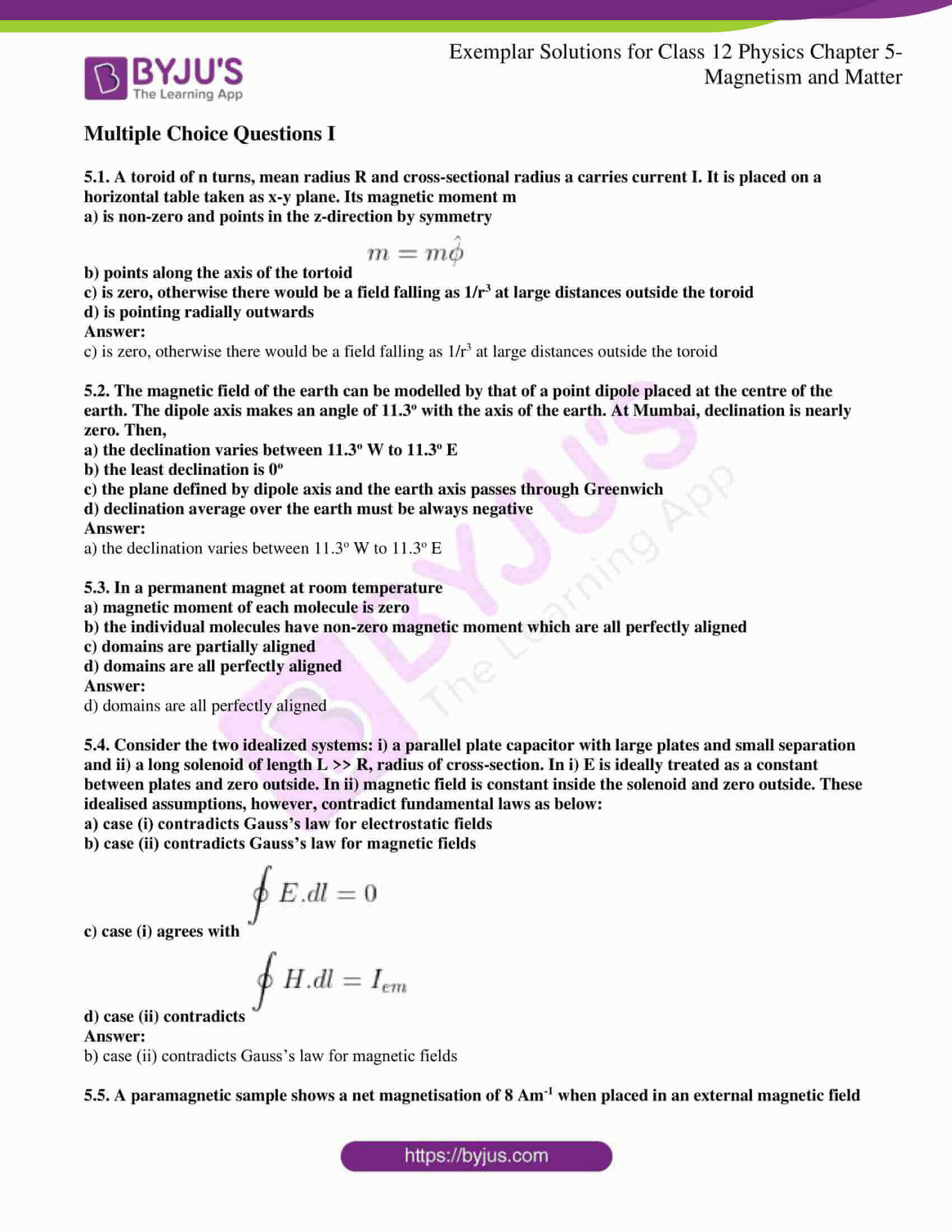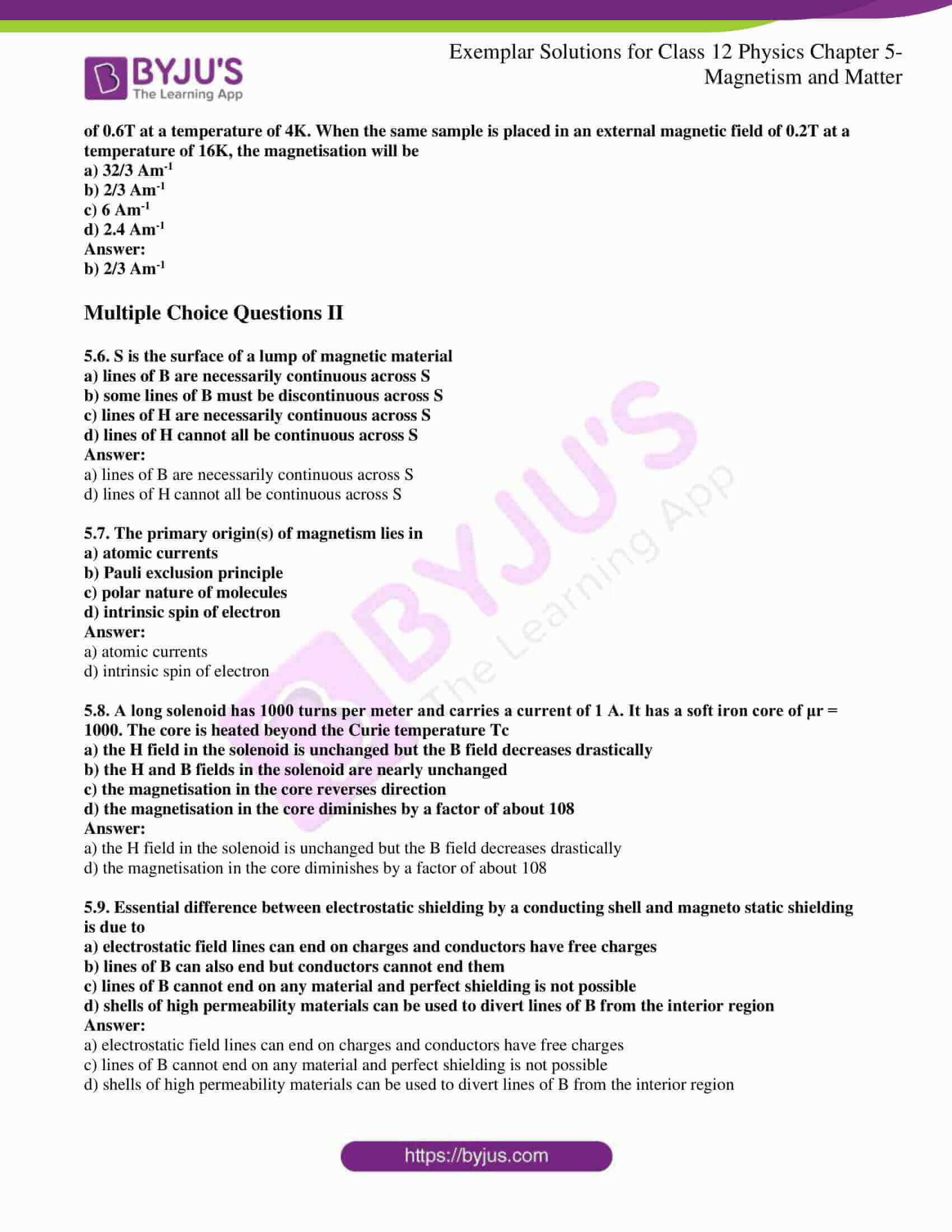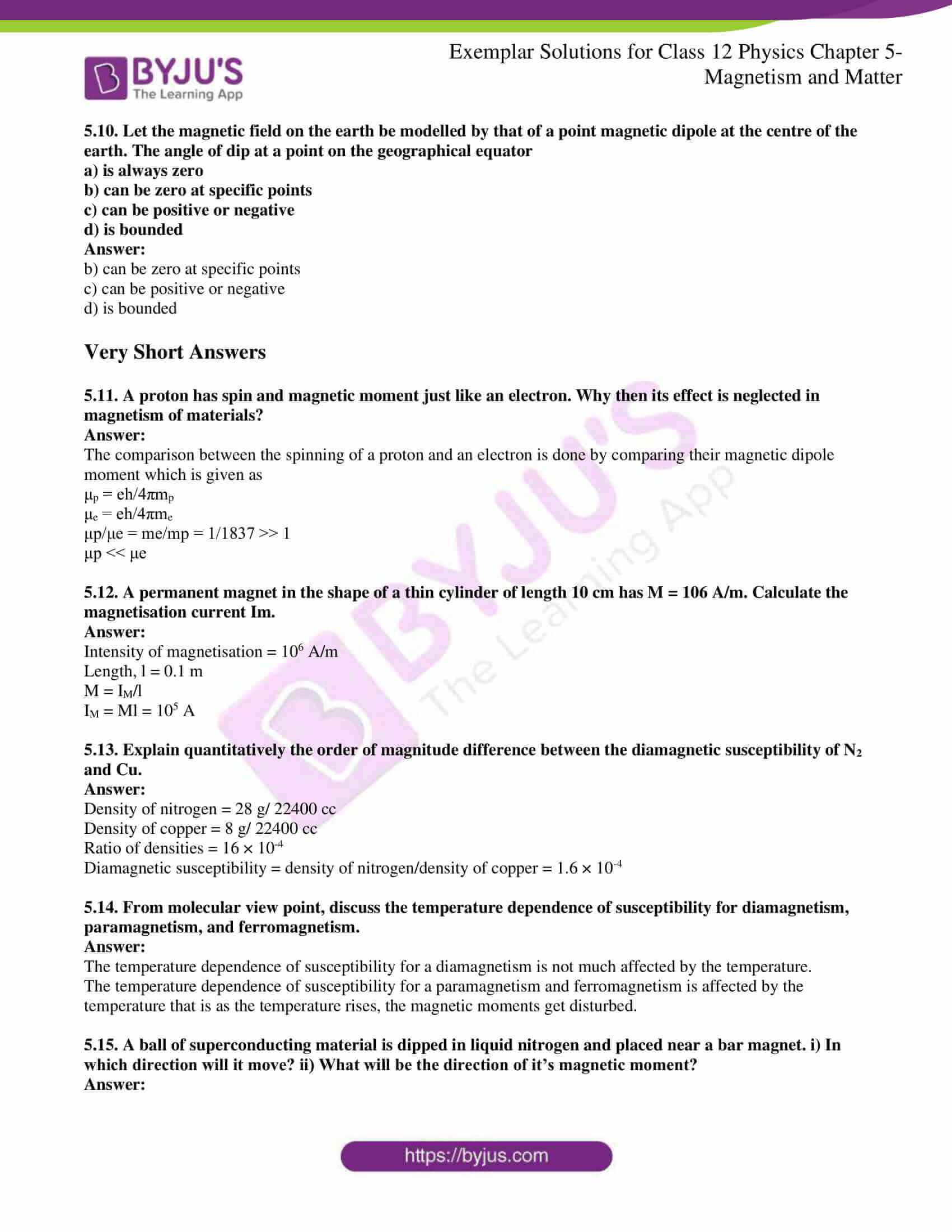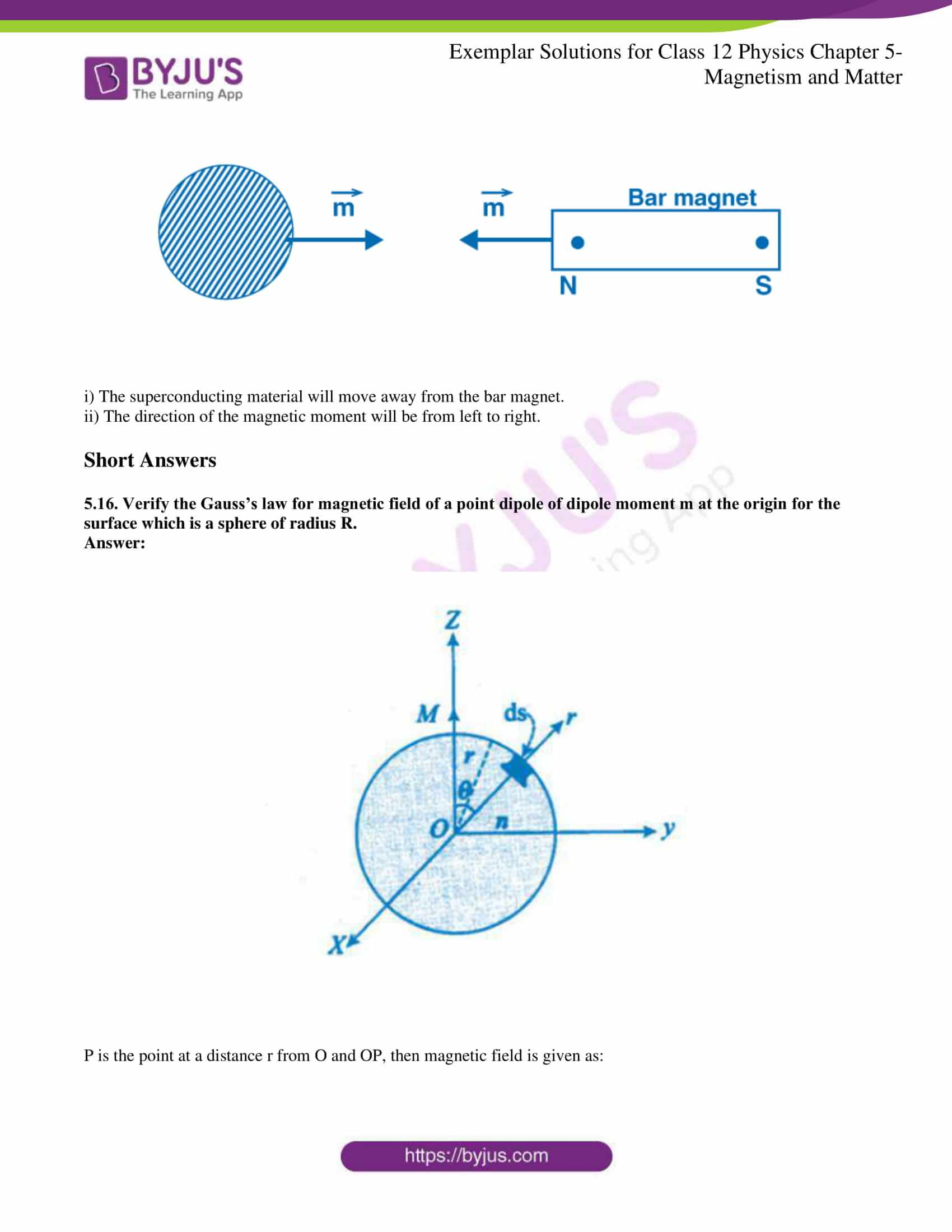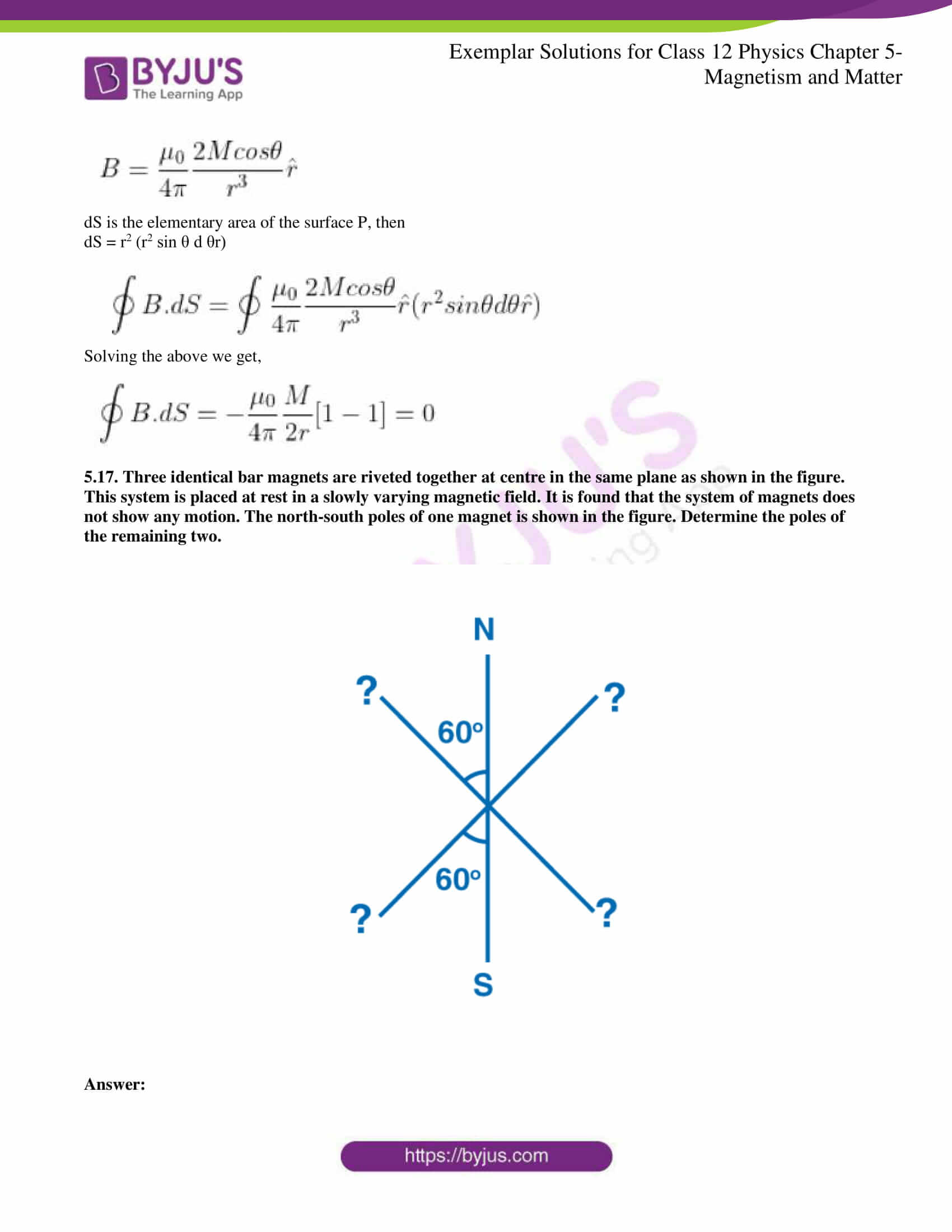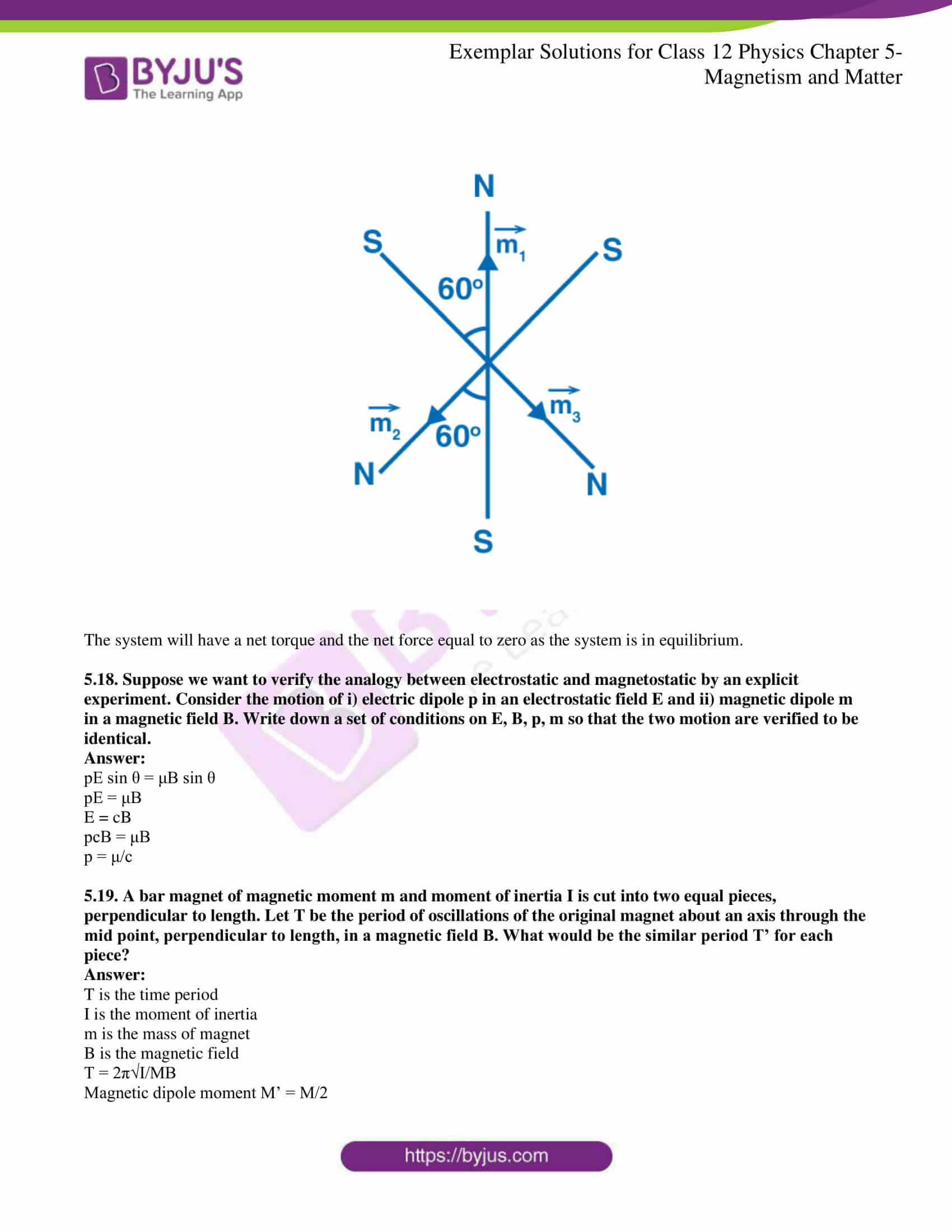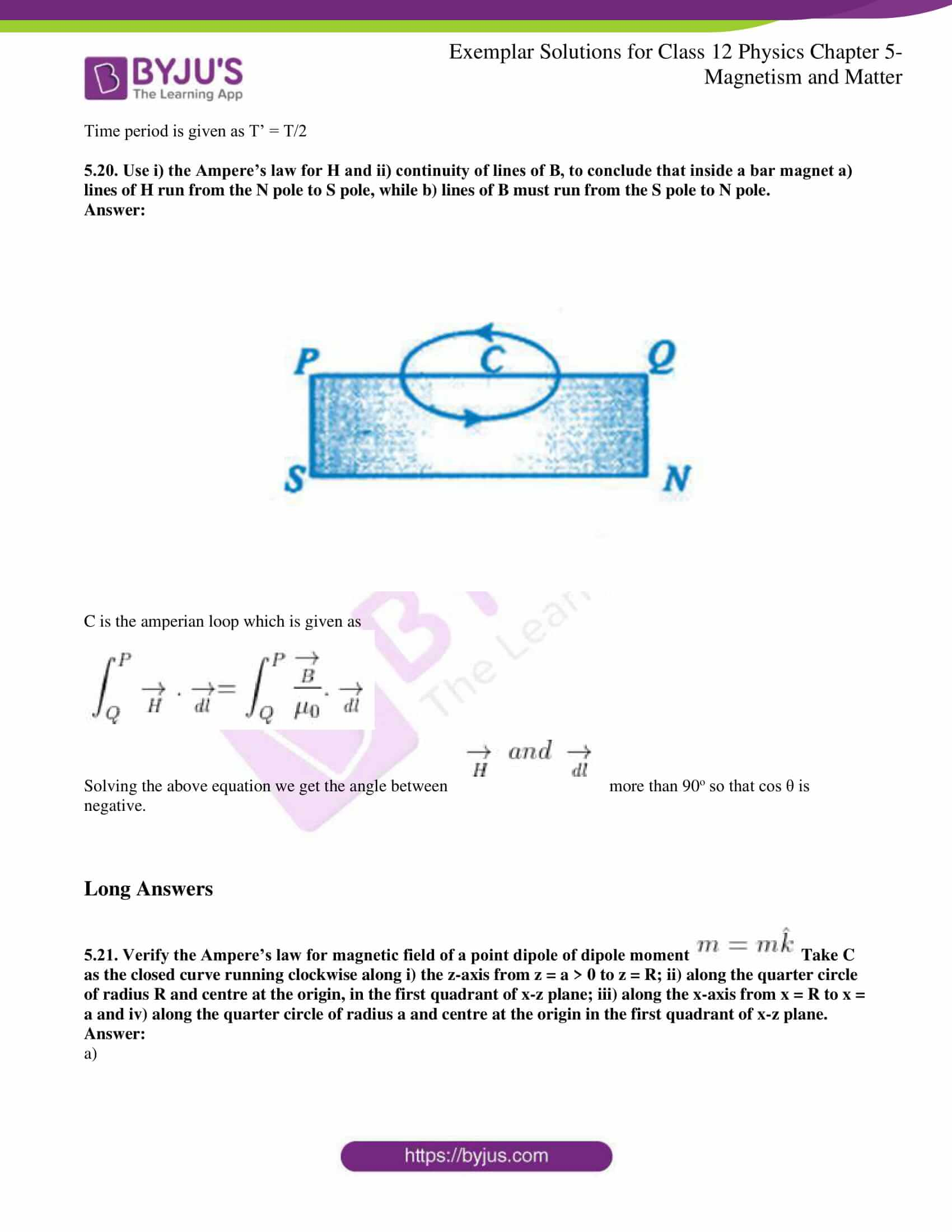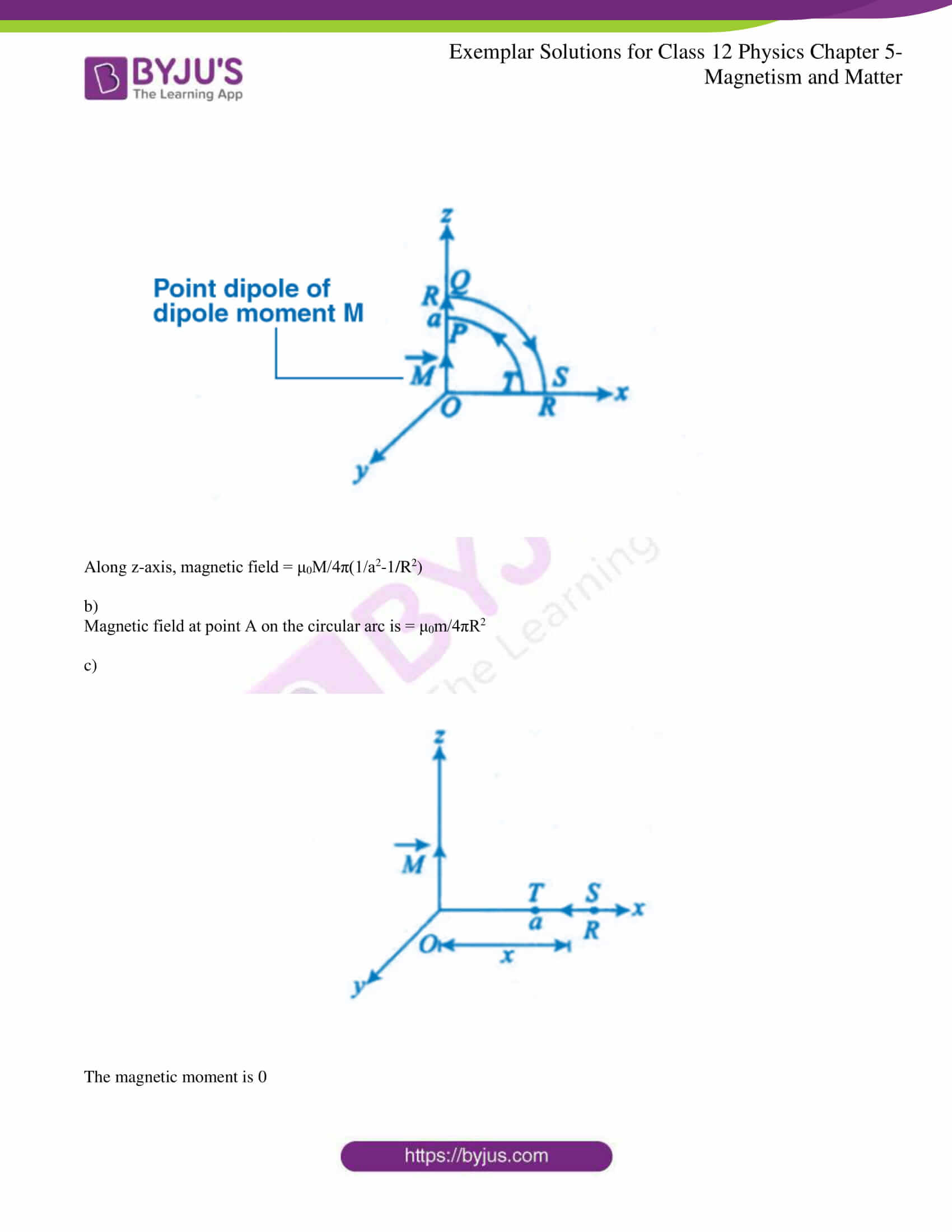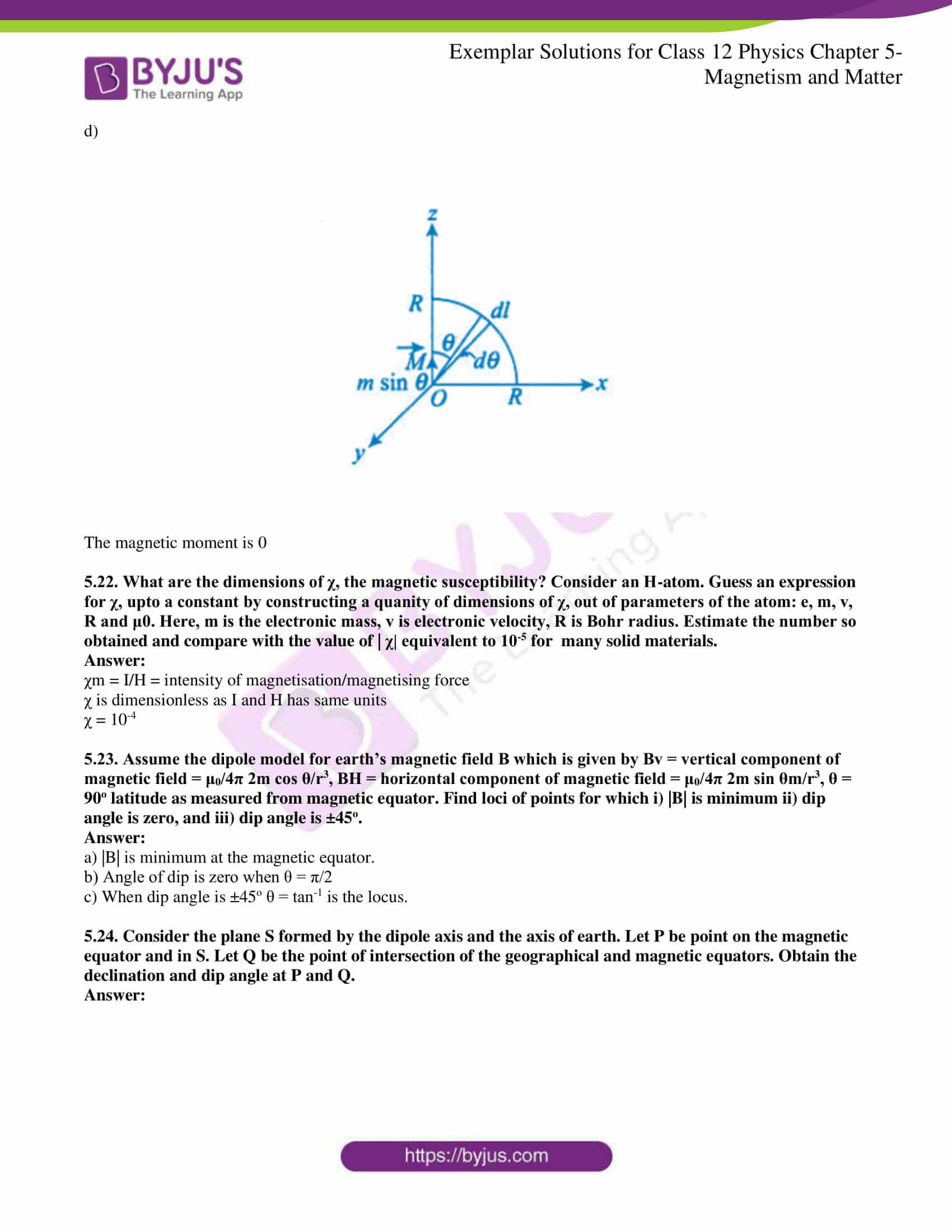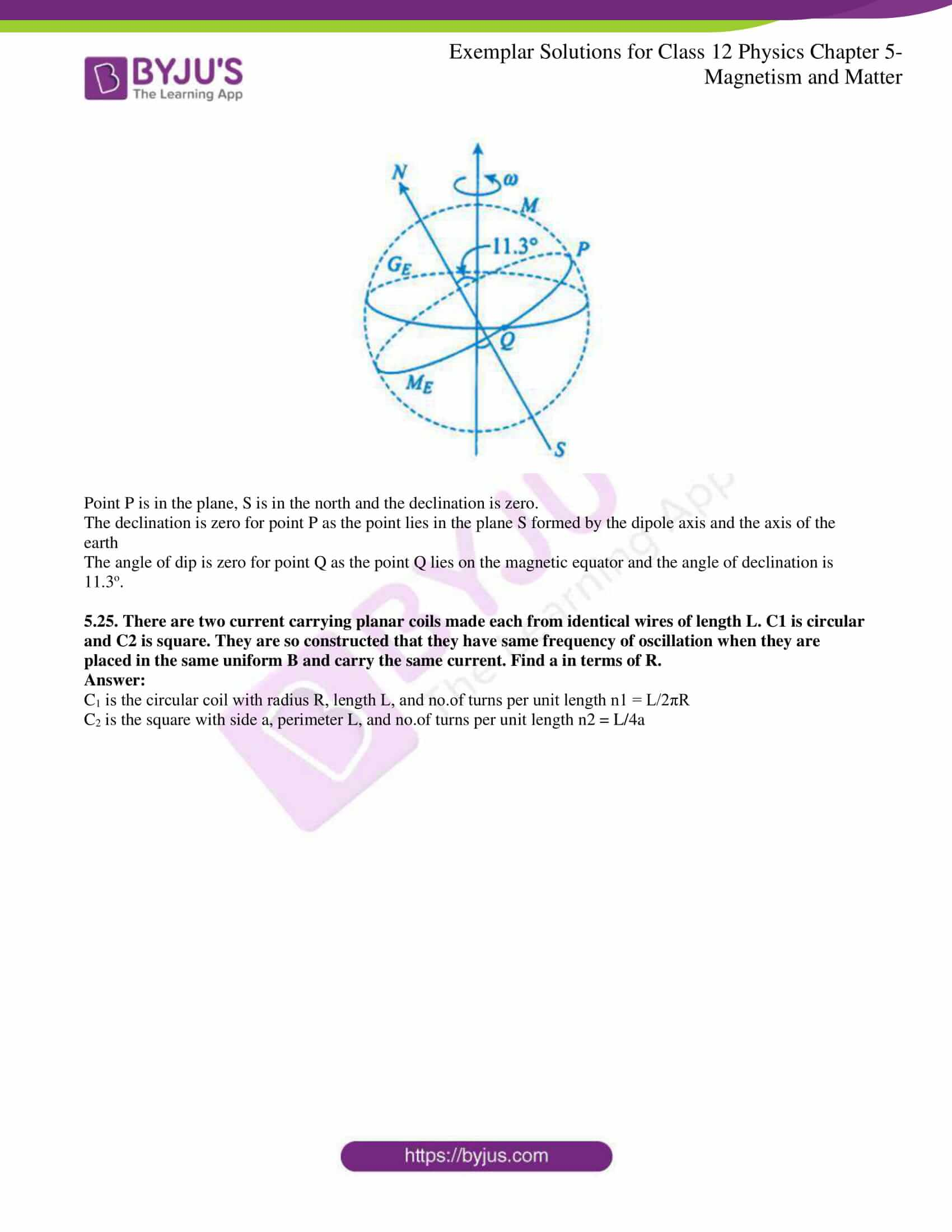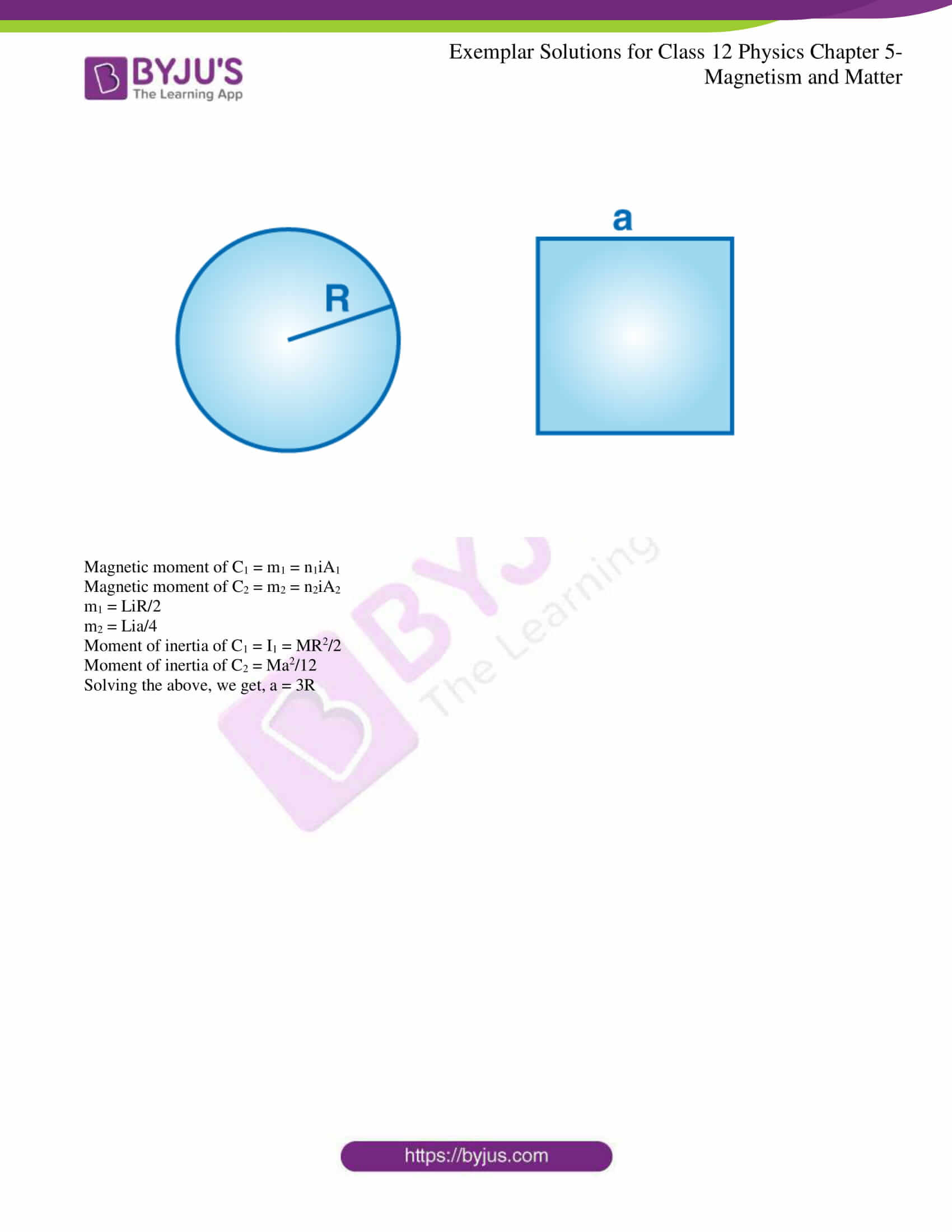### Multiple Choice Questions I

5.1. A toroid of n turns, mean radius R and cross-sectional radius a carries current I. It is placed on a horizontal table taken as an x-y plane. Its magnetic moment m

a) is non-zero and points in the z-direction by symmetry

b) points along the axis of the toroid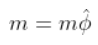c) is zero, otherwise, there would be a field falling as 1/r3 at large distances outside the toroid

c) is zero, otherwise, there would be a field falling as 1/r3 at large distances outside the toroid

5.2. The magnetic field of the earth can be modelled by that of a point dipole placed at the centre of the earth. The dipole axis makes an angle of 11.3o with the axis of the earth. At Mumbai, declination is nearly zero. Then,

a) the declination varies between 11.3o W to 11.3o E

b) the least declination is 0o

c) the plane defined by the dipole axis and the earth axis passes through Greenwich

d) declination average over the earth must always be negative

a) the declination varies between 11.3o W to 11.3o E

5.3. In a permanent magnet at room temperature

a) magnetic moment of each molecule is zero

b) the individual molecules have a non-zero magnetic moment which is all perfectly aligned

c) domains are partially aligned

d) domains are all perfectly aligned

d) domains are all perfectly aligned

5.4. Consider the two idealized systems: i) a parallel plate capacitor with large plates and small separation and ii) a long solenoid of length L >> R, the radius of the cross-section. In i) E is ideally treated as a constant between plates and zero outside. In ii) the magnetic field is constant inside the solenoid and zero outside. These idealised assumptions, however, contradict fundamental laws as below:

a) case (i) contradicts Gauss’s law for electrostatic fields

b) case (ii) contradicts Gauss’s law for magnetic fields

c) case (i) agrees with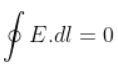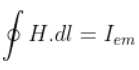b) case (ii) contradicts Gauss’s law for magnetic fields

5.5. A paramagnetic sample shows a net magnetisation of 8 Am-1 when placed in an external magnetic field of 0.6T at a temperature of 4K. When the same sample is placed in an external magnetic field of 0.2T at a temperature of 16K, the magnetisation will be

a) 32/3 Am-1

b) 2/3 Am-1

c) 6 Am-1

d) 2.4 Am-1

b) 2/3 Am-1

### Multiple Choice Questions II

5.6. S is the surface of a lump of magnetic material

a) lines of B are necessarily continuous across S

b) some lines of B must be discontinuous across S

c) lines of H are necessarily continuous across S

d) lines of H cannot all be continuous across S

a) lines of B are necessarily continuous across S

d) lines of H cannot all be continuous across S

5.7. The primary origin(s) of magnetism lies in

a) atomic currents

b) Pauli exclusion principle

c) polar nature of molecules

d) intrinsic spin of electron

a) atomic currents

d) intrinsic spin of electron

5.8. A long solenoid has 1000 turns per meter and carries a current of 1 A. It has a soft iron core of μr = 1000. The core is heated beyond the Curie temperature Tc

a) the H field in the solenoid is unchanged, but the B field decreases drastically

b) the H and B fields in the solenoid are nearly unchanged

c) the magnetisation in the core reverses the direction

d) the magnetisation in the core diminishes by a factor of about 108

a) the H field in the solenoid is unchanged, but the B field decreases drastically

d) the magnetisation in the core diminishes by a factor of about 108

5.9. Essential difference between electrostatic shielding by a conducting shell and magnetostatic shielding is due to

a) electrostatic field lines can end on charges, and conductors have free charges

b) lines of B can also end, but conductors cannot end them

c) lines of B cannot end on any material, and perfect shielding is not possible

d) shells of high permeability materials can be used to divert lines of B from the interior region

a) electrostatic field lines can end on charges, and conductors have free charges

c) lines of B cannot end on any material, and perfect shielding is not possible

d) shells of high permeability materials can be used to divert lines of B from the interior region

5.10. Let the magnetic field on the earth be modelled by that of a point magnetic dipole at the centre of the earth. The angle of dip at a point on the geographical equator

a) is always zero

b) can be zero at specific points

c) can be positive or negative

d) is bounded

b) can be zero at specific points

c) can be positive or negative

d) is bounded

5.11. A proton has a spin and magnetic moment, just like an electron. Then, why is its effect neglected in the magnetism of materials?

The comparison between the spinning of a proton and an electron is done by comparing their magnetic dipole moment, which is given as

μp = eh/4πmp

μe = eh/4πme

μp/μe = me/mp = 1/1837 >> 1

μp << μe

5.12. A permanent magnet in the shape of a thin cylinder of length 10 cm has M = 106 A/m. Calculate the magnetisation current I m.

Intensity of magnetisation = 106 A/m

Length, l = 0.1 m

M = IM/l

IM = Ml = 105 A

5.13. Explain the order of magnitude difference between the diamagnetic susceptibility of N2 and Cu quantitatively.

The density of nitrogen = 28 g/ 22400 cc

The density of copper = 8 g/ 22400 cc

Ratio of densities = 16 × 10-4

Diamagnetic susceptibility = density of nitrogen/density of copper = 1.6 × 10-4

5.14. From a molecular viewpoint, discuss the temperature dependence of susceptibility for diamagnetism, paramagnetism, and ferromagnetism.

The temperature dependence of susceptibility for a diamagnetism is not much affected by the temperature.

The temperature dependence of susceptibility for paramagnetism and ferromagnetism is affected by the temperature that is, as the temperature rises, the magnetic moments get disturbed.

5.15. A ball of superconducting material is dipped in liquid nitrogen and placed near a bar magnet. i) In which direction will it move? ii) What will be the direction of its magnetic moment?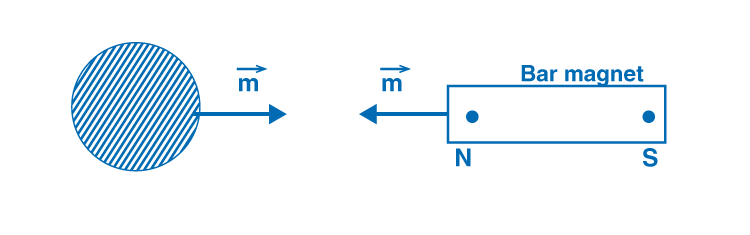i) The superconducting material will move away from the bar magnet.

ii) The direction of the magnetic moment will be from left to right.

5.16. Verify Gauss’s law for the magnetic field of a point dipole of dipole moment m at the origin for the surface, which is a sphere of radius R.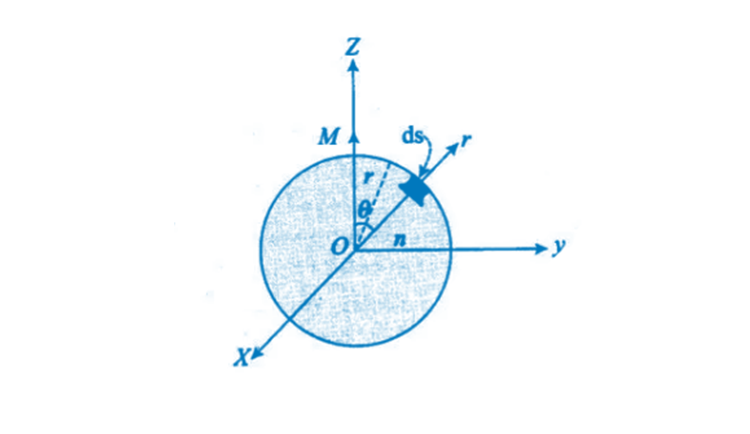P is the point at a distance r from O and OP, then the magnetic field is given as: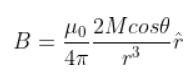dS is the elementary area of the surface P, then

dS = r2 (r2 sin θ d θr)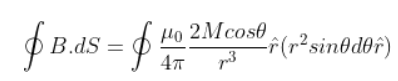Solving the above, we get,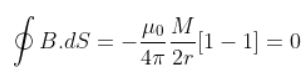5.17. Three identical bar magnets are riveted together at the centre in the same plane, as shown in the figure. This system is placed at rest in a slowly varying magnetic field. It is found that the system of magnets does not show any motion. The north-south poles of one magnet are shown in the figure. Determine the poles of the remaining two.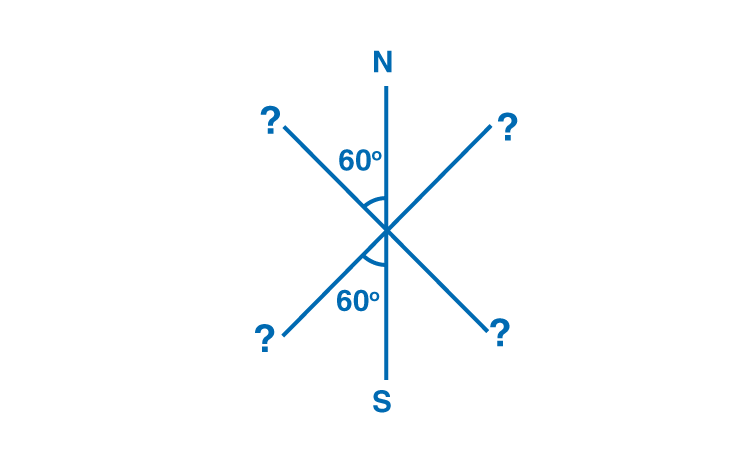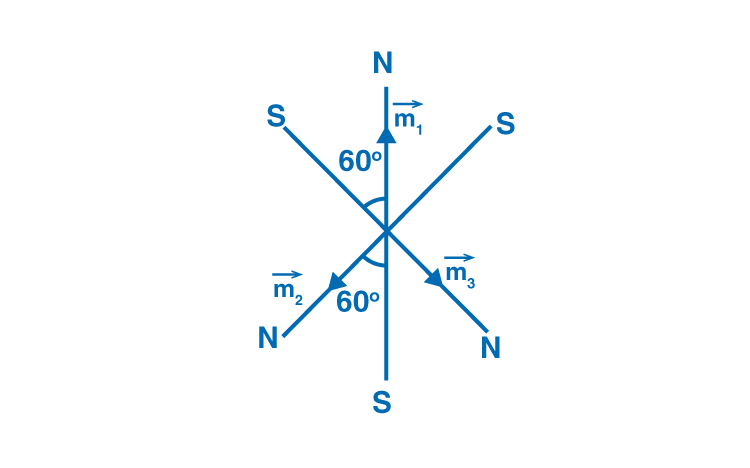The system will have a net torque and the net force equal to zero as the system is in equilibrium.

5.18. Suppose we want to verify the analogy between electrostatic and magnetostatic by an explicit experiment. Consider the motion of i) electric dipole p in an electrostatic field E and ii) magnetic dipole m in a magnetic field B. Write down a set of conditions on E, B, p, m so that the two movements are verified to be identical.

pE sin θ = μB sin θ

pE = μB

E = cB

pcB = μB

p = μ/c

5.19. A bar magnet of magnetic moment m and moment of inertia I are cut into two equal pieces, perpendicular to the length. Let T be the period of oscillations of the original magnet about an axis through the midpoint, perpendicular to the length, in a magnetic field B. What would be the similar period T’ for each piece?

T is the time period

I is the moment of inertia

m is the mass of the magnet

B is the magnetic field

T = 2π√I/MB

Magnetic dipole moment M’ = M/2

The time period is given as T’ = T/2

5.20. Use i) the Ampere’s law for H and ii) the continuity of lines of B to conclude that inside a bar magnet, a) lines of H run from the N pole to the S pole, while b) lines of B must run from the S pole to N pole.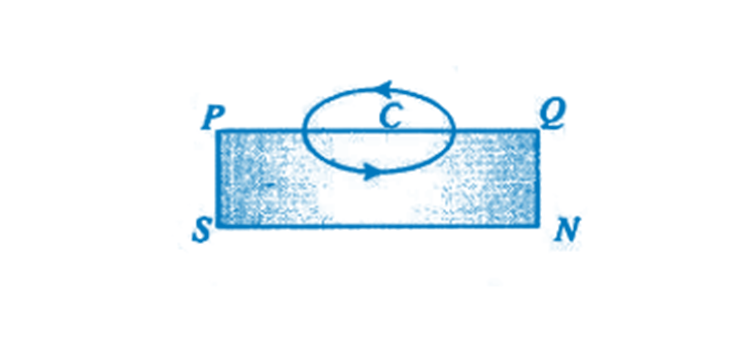C is the Amperian loop which is given as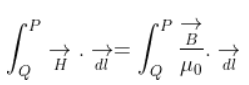Solving the above equation, we get the angle between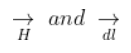more than 90o so that cos θ is negative.

5.21. Verify the Ampere’s law for the magnetic field of a point dipole of dipole moment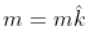Take C as the closed curve running clockwise along i) the z-axis from z = a > 0 to z = R; ii) along the quarter circle of radius R and centre at the origin, in the first quadrant of x-z plane; iii) along the x-axis from x = R to x = a and iv) along the quarter circle of radius a and centre at the origin in the first quadrant of the x-z plane.

a)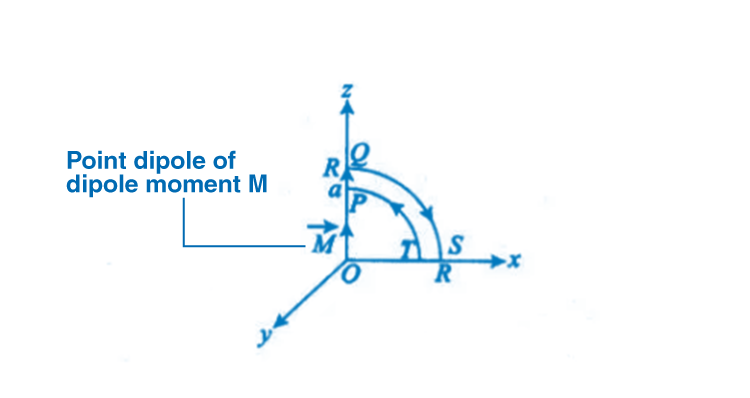Along z-axis, magnetic field = μ0M/4π(1/a2-1/R2)

b)

The magnetic field at point A on the circular arc is = μ0m/4πR2

c)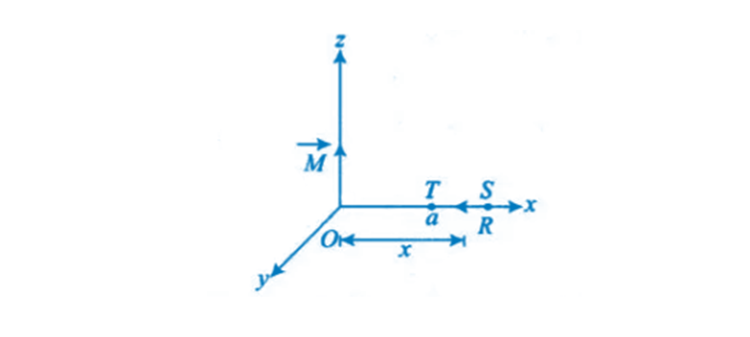The magnetic moment is 0

d)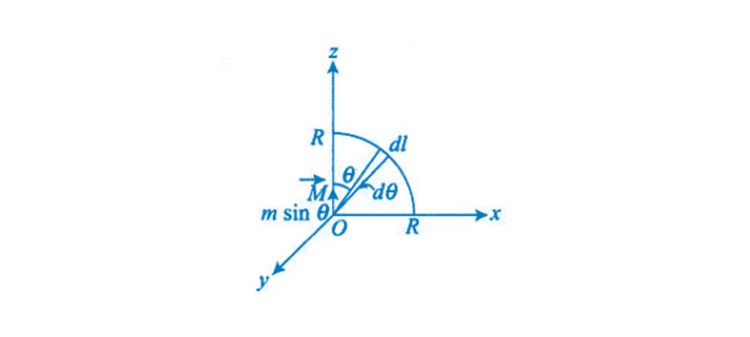The magnetic moment is 0

5.22. What are the dimensions of χ, the magnetic susceptibility? Consider an H-atom. Guess an expression for χ, up to a constant, by constructing a quantity of dimensions of χ, out of parameters of the atom: e, m, v, R and μ0. Here, m is the electronic mass, v is electronic velocity, and R is Bohr radius. Estimate the number so obtained and compare it with the value of | χ| equivalent to 10-5 for many solid materials.

χm = I/H = intensity of magnetisation/magnetising force

χ is dimensionless as I and H have the same units

χ = 10-4

5.23. Assume the dipole model for earth’s magnetic field B, which is given by Bv = vertical component of magnetic field = μ0/4π 2m cos θ/r3, BH = horizontal component of magnetic field = μ0/4π 2m sin θm/r3, θ = 90o latitude as measured from magnetic equator. Find loci of points for which i) |B| is minimum, ii) dip angle is zero, and iii) dip angle is ±45o.

a) |B| is the minimum at the magnetic equator.

b) Angle of dip is zero when θ = π/2

c) When dip angle is ±45o θ = tan-1 is the locus.

5.24. Consider the plane S formed by the dipole axis and the axis of the earth. Let P be a point on the magnetic equator and in S. Let Q be the point of intersection of the geographical and magnetic equators. Obtain the declination and dip angle at P and Q.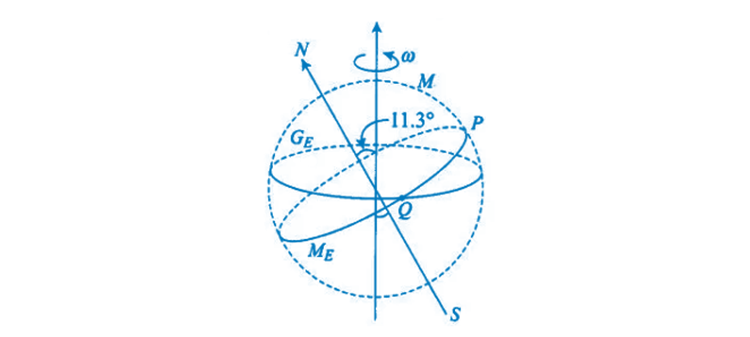Point P is in the plane, S is in the north, and the declination is zero.

The declination is zero for point P as the point lies in the plane S formed by the dipole axis and the axis of the earth

The angle of dip is zero for point Q as point Q lies on the magnetic equator, and the angle of declination is 11.3o.

5.25. There are two current-carrying planar coils made each from identical wires of length L. C1 is circular, and C2 is square. They are so constructed that they have the same frequency of oscillation when they are placed in the same uniform B and carry the same current. Find a in terms of R.

C1 is the circular coil with radius R, length L, and no.of turns per unit length n1 = L/2πR

C2 is the square with side a, perimeter L, and no.of turns per unit length n2 = L/4a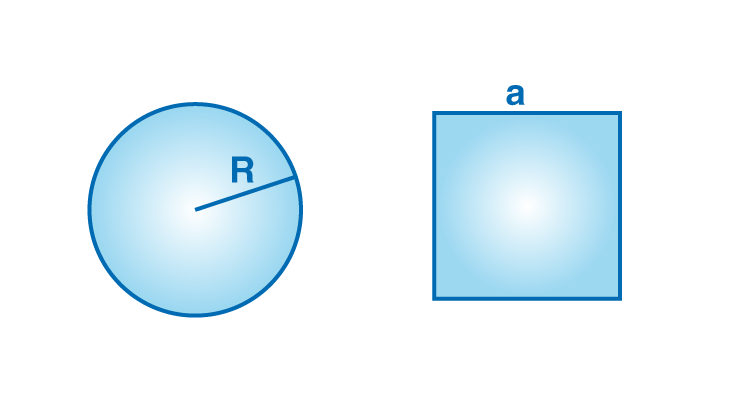Magnetic moment of C1 = m1 = n1iA1

Magnetic moment of C2 = m2 = n2iA2

m1 = LiR/2

m2 = Lia/4

Moment of inertia of C1 = I1 = MR2/2

Moment of inertia of C2 = Ma2/12

Solving the above, we get a = 3R

Magnetism is a property which explains the phenomenon of two interactive objects which are magnetic in nature and portray the virtue of an attractive or repulsive force. The electrons in an atom move in a certain motion, leading to the phenomenon of magnetism occurring.

Important concepts covered in Chapter 5, Magnetism and Matter, are listed below:

1. Magnetism Introduction
2. Bar Magnet – formula derivation and problems
1. The magnetic field lines
2. Bar magnet as an equivalent solenoid
3. The dipole in a uniform magnetic field
4. The electrostatic analogue>
3. Magnetism and Gauss’s Law – Statement, derivation, numerical
4. The Earth’s Magnetism
1. Magnetic declination and dip
5. Magnetisation and Magnetic Intensity – Net magnetic moment per unit volume derivation and calculation
6. Magnetic Properties of Materials
1. Diamagnetism
2. Paramagnetism
3. Ferromagnetism

Practise the NCERT Exemplar Solutions for Class 12 Physics Chapter 5, Magnetism and Matter, by downloading the PDFs given above.

The study materials provided on BYJU’S website are the result of thorough study and research by subject experts having vast experience in the respective field. They help students to understand the concepts thoroughly without leaving any doubts. In order to get good marks in the board exam, students are advised to solve previous years’ question papers and sample papers.